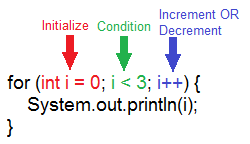# For Loops

For loops allow us to repeat code a fixed number of times. A basic for loop is structured like this:

``````for (int i = 0; i < COUNT; i++)
{
// Code segment that is executed COUNT times.
}
``````

## Breaking Down the For Loop

A for loop can be divided into three major parts. The first part initializes the loop variable, the second part tests some condition, and the third part increments or decrements the loop variable. Each part of the for loop is separated by a semicolon (`;`).

Let's break down the following example into its three major parts:#### Part 1: Initialize

The first part of a for loop initializes some variable. In the example above, `int i = 0` initially sets the variable `i` equal to `0`.

#### Part 2: Condition

The second part of a for loop tests a condition. If the condition is true, the code within the for loop executes. If the condition is false, the code within the for loop is skipped. In the example above, i < 3 compares the current value of our variable `i` to determine if it is less than `3`. Each time `i` is less than `3`, the value of `i` will be printed to the screen. This happens three times.

#### Part 3: Increment/Decrement

The third part of a for loop changes the variable after each time the loop runs. Remember, `i++` means 1 is added to `i`. It is incremented. Conversely, `i--` means 1 is subtracted from `i`. It is decremented.

#### Putting It All Together

What happens when we run the example for loop? What gets printed to the screen?

The first part of our for loop, `int i = 0`, initializes our variable. It sets our loop variable `i` equal to `0` Next, the condition part of the loop is evaluated. Is i < 3? Since `i` is currently set to `0`, and `0` is indeed less than `3`, the condition i < 3 is true. Thus, the code within the for loop is executed. `0` is printed to the screen. After this, `i` is incremented. `i++` means we add 1 to `i`, so `i` is now set to `1`.

Since `i` is now set to `1`, the condition i < 3 is re-evaluated. It is still true. `1` is now printed to the screen. `i` is incremented again and becomes set to `2`.

The condition i < 3 is re-evaluated. Since `i` is now set to `2`, our condition is still true. `2` is printed to the screen. `i` is incremented again and becomes set to `3`.

Finally, when the condition i < 3 is re-evaluated, our condition is false. 3 is not less than 3, so the code within the for loop is skipped. Nothing else gets printed. The for loop is done.

Thus, our output would be:

``````0
1
2
``````

## More Examples

### Countdown

In this countdown example, `i` is initially set to 5. We decrement (or subtract) 1 from `i` on each iteration of the for loop. We print out each value of `i` until it reaches 0.

``````public class Countdown extends ConsoleProgram
{
public void run()
{
System.out.println("Initiating countdown:");
for(int i = 5; i >= 0; i--)
{
System.out.println(i + "...");
}
}
}
``````

Output:

``````Initiating countdown:
5...
4...
3...
2...
1...
0...
``````

### Count by Twos

Instead of incrementing or decrementing `i` by only 1, we will increment `i` by adding 2 in this example instead. This allows us to count up by two each time.

``````public class CountByTwos extends ConsoleProgram
{
public void run()
{
for(int i = 0; i < 21; i += 2)
{
System.out.println(i);
}
}
}
``````

Output:

``````0
2
4
6
8
10
12
14
16
18
20
``````

### For Loop Sum Program

Here is a program which sums all the numbers from 1 to 100. The for loop adds the numbers 1+2+3+4+5+6+.....+98+99+100. Since this program uses global constants, we can easily change the `MIN` and `MAX` values used in our sum without having to touch the for loop at all.

``````public class ForLoopSum extends ConsoleProgram
{
/* MIN and MAX are constants. Constants are variables that do not change.
their purposes later. */
private static final int MIN = 1;
private static final int MAX = 100;

// This program adds the numbers from 1 to 100.
public void run()
{
int sum = 0;
for(int i = MIN; i < = MAX; i++)
{
sum += i;
}
System.out.println("The sum was " + sum);
}
}
``````

Output:

``````The sum was 5050
``````